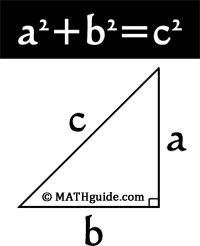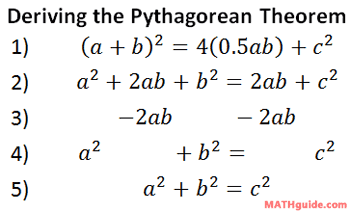Pythagorean Theorem
 Home > Lessons > Pythagorean Theorem Search | Updated February 22nd, 2019
Introduction

The Pythagorean Theorem is a mathematical relationship between the sides of a right triangle. A right triangle is any triangle that has one right internal angle. Pythagoras stated, if the length of the legs (smallest side) are squared and their sum is found, the sum will be equal to the square of the hypotenuse (longest side). Algebraically speaking, the relationship looks like...The Pythagorean Theorem

The legs are traditionally marked with an 'a' and a 'b,' while the hypotenuse is marked with a 'c.' If that is the case, then the formula is a2 + b2 = c2. The next section will explain how the equation can be derived.

The derivation of the algebraic relationship is also not very complicated, as far as most derivations go.

To derive it, we will examine the diagram below. The diagram consists of many simple figures. There is a white rotated square at the center. There are four blue right triangles. The entire figure, the rotated square and the four right triangles, is a large square, too."Pythagorean Squares"

The following explanation requires knowledge of area. Specifically, the areas of rectangles and triangles must be known to understand the explanation. Review the areas of the two figures before progressing.

The area of the inner white square is: A = (c)(c) = c2. The area of one blue right triangle is: A = (a)(b)÷2 = 0.5ab. The area of the entire square, which includes the white rotated square and the blue right triangles, is: A = (a+b)2. The derivation below begins with these areas in step one.• Step One:
The left side of the equation is the area of the largest square [(a+b)2]. The right side represents the area of the smallest square [c2] and the area of four right triangles [4(0.5ab)]. The sum of the area of the small parts is equal to the area of the entire figure.
• Step Two:
The left side of the equation is the result of expanding the binomial (a+b)2 or multiplying (a+b)(a+b) = a2 + ab + ab + b2 = a2 + 2ab + b2. The right side results when 4(0.5) is simplified to 2.
• Step Three:
The value 2ab is subtracted from both sides of the equation, which cancels the term from both side of the equation.
• Step Four:
The remaining values are left behind.
• Step Five:
Collecting the terms results in the familiar formula, The Pythagorean Theorem.

This means: "the sum of the squares of the legs of a right triangle is equal to the square of the hypotenuse." Watch our video that fully explains this proof of The Pythagorean Theorem.ideo: Proof of The Pythagorean Theorem

You may want to use these activities that scaffold a step-by-step process for proving the Pythagorean Theorem.ctivity: Proving the Pythagorean Theorem (common method)ctivity: Proving the Pythagorean Theorem (President Garfield's method)

The sections below will indicate how to use the relationship for two situations. One section tell us how to find a missing hypotenuse and the other a missing leg.

This section will explain how to use The Pythagorean Theorem to find a missing hypotenuse. If we are given a triangle's legs, then we would use the equation to calculate the length of the hypotenuse. Say we know the shortest sides to be 3 m and 7 m. The example below outlines the process by which we can calculate the hypotenuse.1. The equation is written. This will allow us to organize our information and process it accordingly.
2. The values for 'a' and 'b' are placed within the equation, since they represent the lengths of the shortest sides.
3. Order of operations dictates that the values should be squared before they are added. 3 x 3 = 9 and 7 x 7 = 49.
4. The sum of 9 and 49 is 58, or 9 + 49 = 58.
5. To cancel the square of c, the right side of the equation, we must take the square root of both sides. The square root of a square of a value is equal to the original value. If we must take the square root of the right side, the same must be done to the left side.
6. The square root of 58 is 7.6157. Only four decimal places of an infinitely long, non-repeating answer has been displayed.
7. The final answer has been rounded to the hundredths place. Since the thousandths place was five or greater, the hundredths place was increased by 1.

Since our final value for c is 7.62, it means that the hypotenuse is 7.62 m in length. It also means we are done with the example.ideo: How to Use the Pythagorean Theorem: Hypotenuseuizmaster: Find the Length of a Hypotenuse Given Two Sides

This section will explain how to use The Pythagorean Theorem to find a missing leg. If we are given a triangle's leg and hypotenuse, then we would use the equation to calculate the length of the missing leg. Say we know the longest length to be 11 in and one of the other shorter sides to be 6 in. The example below outlines the process by which we can calculate the missing length.1. The equation is written.
2. The letter 'c' is replaced by the length of the longest side, 11 in. The letter 'a' is replaced by 6 in. [Since we know the longest side is 11 in, we could have replaced the letter 'b' with 6. We would still arrive at the same solution, which is left for independant investigation.]
3. The values are squared. 6 x 6 = 36 and 11 x 11 = 121.
4. To get the unknown value alone, we need to subtract 36 from both sides of the equation.
5. b2 is the value left on the left side. 121 - 36 = 85, and that is the remaining value on the right side.
6. To cancel the square and obtain the value of 'b,' we must cancel the square root. To cancel the square, we must take the square root. The same must be done to the other side, as well.
7. The square root of 85 is 9.2195. Four decimal places have been shown even though the true value has an infinite number of non-repeating decimal values.
8. The value has been rounded to the nearest tenth. Since the hundredth value is less than five, the number is reported up to the first decimal value.

Since our final value for a is 9.2, it means that the missing leg is 9.2 inches in length. It also means we are done with the example.ideo: How to Use the Pythagorean Theorem: Leguizmaster: Find a Leg Given the Hypotenuse and the Other Leg

 Videos Watch these instructional videos.ideo: How to Use the Pythagorean Theorem: Hypotenuseideo: How to Use the Pythagorean Theorem: Leg Quizmasters After reading the lessons, try our quizmasters. MATHguide has developed numerous testing and checking programs to solidify these skills:uizmaster: Find the Length of a Hypotenuse Given Two Sidesuizmaster: Find a Leg Given the Hypotenuse and the Other Leg Activities You may want to use this activity that scaffolds the step-by-step process for proving the Pythagorean Theorem.ctivity: Proving the Pythagorean Theorem Related Lessons Here are lessons related to the Pythagorean Theorem.esson: Diagonal Length of a Cubeesson: Coordinate Geometry (see Distance Formula)esson: Conic Sections (see Foci)esson: Trigonometry with Right Trianglesesson: Law of Cosinesesson: Trigonometric Expressionsesson: Vectorsesson: Polar Coordinates